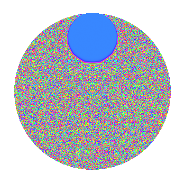# Properties

 Label 3.71.bLevel 3 Weight 71 Character orbit b Rep. character $$\chi_{3}(2,\cdot)$$ Character field $$\Q$$ Dimension 22 Newform subspaces 1 Sturm bound 23 Trace bound 0

# Related objects

## Defining parameters

 Level: $$N$$ $$=$$ $$3$$ Weight: $$k$$ $$=$$ $$71$$ Character orbit: $$[\chi]$$ $$=$$ 3.b (of order $$2$$ and degree $$1$$) Character conductor: $$\operatorname{cond}(\chi)$$ $$=$$ $$3$$ Character field: $$\Q$$ Newform subspaces: $$1$$ Sturm bound: $$23$$ Trace bound: $$0$$

## Dimensions

The following table gives the dimensions of various subspaces of $$M_{71}(3, [\chi])$$.

Total New Old
Modular forms 24 24 0
Cusp forms 22 22 0
Eisenstein series 2 2 0

## Trace form

 $$22q - 57760946614928610q^{3} - 12924724784889679105472q^{4} - 2542746418602623176312578240q^{6} + 3460300354929279082139211020q^{7} - 3187627653443587997840864868739482q^{9} + O(q^{10})$$ $$22q - 57760946614928610q^{3} - 12924724784889679105472q^{4} - 2542746418602623176312578240q^{6} + 3460300354929279082139211020q^{7} - 3187627653443587997840864868739482q^{9} - 204904054456738768728763726683461760q^{10} + 168216518978261501059545818584280696640q^{12} - 1425273500899614630088058291006011908100q^{13} - 225789500492205687292660149319768298715840q^{15} + 7791108876170200924142434286561643963000832q^{16} - 159240712078782992086911930584730978792685440q^{18} + 2012529521265601397979311193864856923750823676q^{19} - 5316334525000974787771605720411010154937122916q^{21} - 177999664576757911046568099334764110597720653440q^{22} + 2221044214363105865421794285118261395337437583360q^{24} - 47913993180689593092577485889381363779493774325690q^{25} - 327350510975933028532160361074129063579708328041490q^{27} + 199622305967867855771313967200368144722234985321600q^{28} + 19529370934845391692884101303550253186745064711003520q^{30} + 12388430374073163692752061441697409777651574405007404q^{31} + 91609538538011962010378972773658529100477255901421120q^{33} + 605761954772905142742127812440233067564260993651653120q^{34} + 903849673866118209515044215587923561361275075218434112q^{36} - 2942122339532414491328953596359839081620064777882528420q^{37} - 83642273913756481299940648535011188445494335818507646004q^{39} + 272642123044960747425938890972217844718938356564315934720q^{40} + 1560147154093916143029768098476489647357578674968337342080q^{42} - 4545359228128189931707988293083620465330530163336481972580q^{43} - 13684191484560547979485162889557225553655737227535310368640q^{45} + 34824945738150746982031713560272687284036228683298922087680q^{46} + 187018310806180504680614747073364302422042767094159794882560q^{48} + 537429667187009430823177633570141302055322425376808156541794q^{49} + 93003444735225900297901054333392923286830613776157770499840q^{51} - 256980181653870794210710907095882776189154704275967737138560q^{52} + 563860949077651296335542553968680463677661322067851314468800q^{54} - 3516118540339332578884347645630886351467431915082786354698880q^{55} + 62619566495727497029128246919971991949893526891401194293093580q^{57} + 116675137250817318744043251484707490614825810469582794383621760q^{58} - 289520688194704548710930657842220413516071423272166989563023360q^{60} - 1004518379975789938184755460502459521248398274882979931600708676q^{61} + 2259750912371699320668016184778015293200259463243321554460021420q^{63} - 6990282608978901236062382909358427229318771679854621867713298432q^{64} + 9802144175872303219237439174047894300074716767326740733265952640q^{66} - 9530737568144236105810003684427263525901520593033925660073496260q^{67} + 68660923095126616358914910008665108044130343377859227725343530880q^{69} - 186825484366209931701114245951001364013348939360625786570907971840q^{70} + 800859191695150280430684829106757981081528103077118327934086410240q^{72} - 498564607593670758195755796246723418636521686724391030404386208820q^{73} + 2109806600351472862056557154002428953234561707550117711339382623630q^{75} - 5263274864463875706518271175142614969672638525330024503218962553216q^{76} + 12308167524701892969963937648343555852922730564344320620076689564800q^{78} - 17307670437682441097149414568687707748355067787533584779481303522964q^{79} + 13169506853202980530135359556542777642532240836639689883271737852342q^{81} - 39431820989895068684167150578955043187025954212565254141576902204160q^{82} + 138763489413867144231869861916118201044140663071190925822310630686336q^{84} - 61037539079877371876604729964700889725410552523647838067356520814080q^{85} - 149627312260702067527556548128053547148656320722965526646581032129600q^{87} + 423107204692289659768926623144292819592688368886126043795359525621760q^{88} - 954822316211616625813572408526438784570624253142422549351495971955840q^{90} + 1351237435163715752950727836854267043101210025128484375869461920848568q^{91} - 1419126662189890378883596636164605113800863195507982624574295260938180q^{93} + 10138980254856605941232671681032460690110853357766177455228626662453760q^{94} - 12383253221007478242150050518024370091385337450937902244753056045137920q^{96} + 5943877415032019678068302054256035030995353737176853716379501144663980q^{97} - 21983160871333538547745741193506138170867254293489738303957813119108480q^{99} + O(q^{100})$$

## Decomposition of $$S_{71}^{\mathrm{new}}(3, [\chi])$$ into newform subspaces

Label Dim. $$A$$ Field CM Traces $q$-expansion
$$a_2$$ $$a_3$$ $$a_5$$ $$a_7$$
3.71.b.a $$22$$ $$93.095$$ None $$0$$ $$-5\!\cdots\!10$$ $$0$$ $$34\!\cdots\!20$$

## Hecke characteristic polynomials

There are no characteristic polynomials of Hecke operators in the database